# Selina Solutions Concise Mathematics Class 6 Chapter 28 Polygons

Selina Solutions Concise Mathematics Class 6 Chapter 28 Polygons has answers created by experts who have vast knowledge in the education industry. A Polygon is a closed plane figure bounded by at least three line segments. Regular practice of solutions helps students to improve time management skills, which are important in attempting examinations. For in-depth knowledge about the concepts covered, students can use Selina Solutions Concise Mathematics Class 6 Chapter 28 Polygons PDF, from the links which are given here.

Chapter 28 Polygons has detailed answers for each and every question relying on Polygons. Students who follow solutions obtain proficiency in solving complex problems effortlessly. This also helps them to build up interest in the respective subjects.

## Selina Solutions Concise Mathematics Class 6 Chapter 28 Polygons Download PDF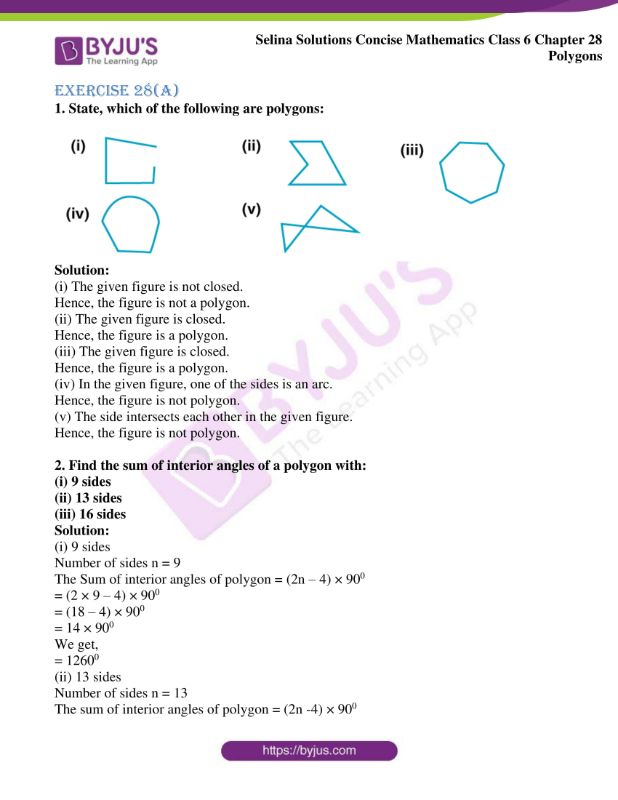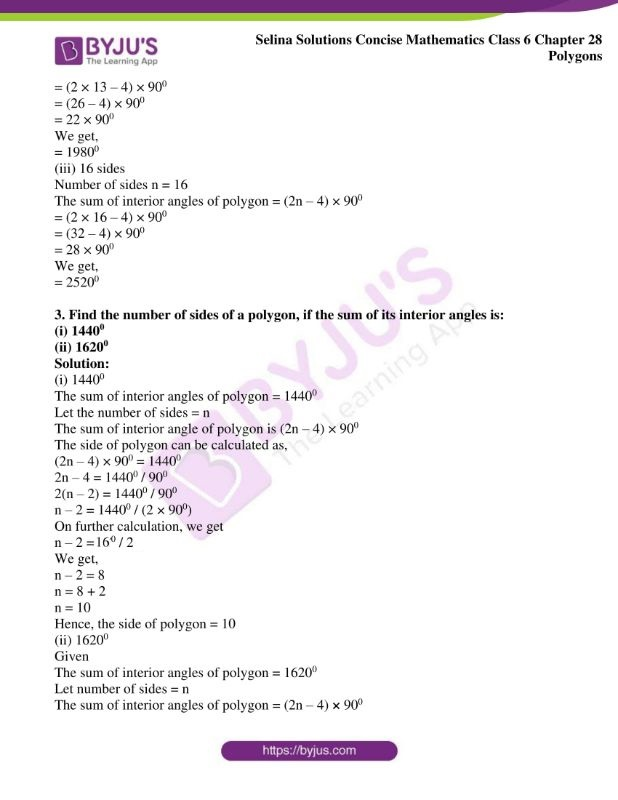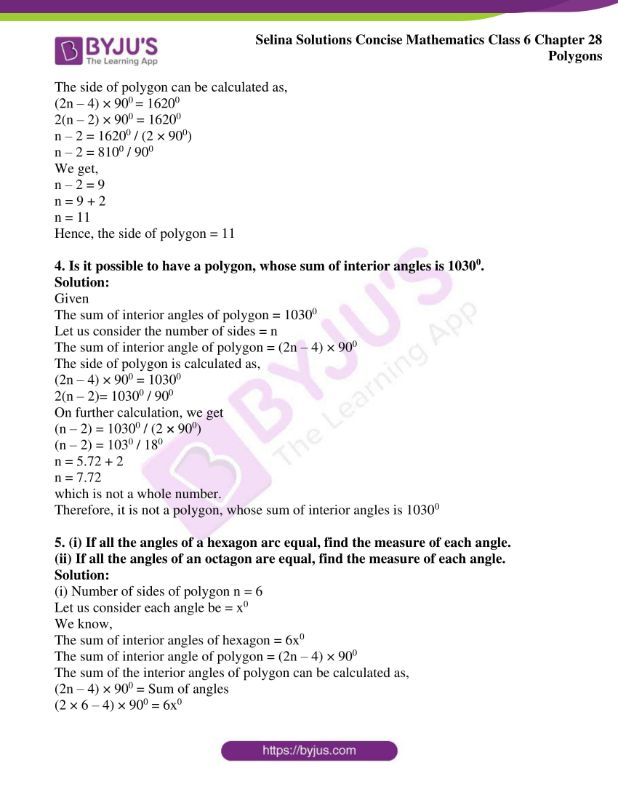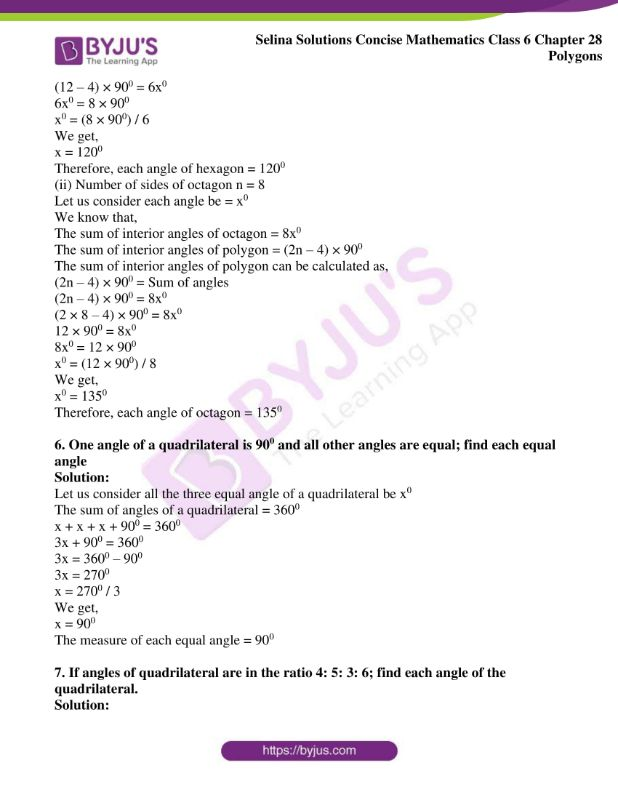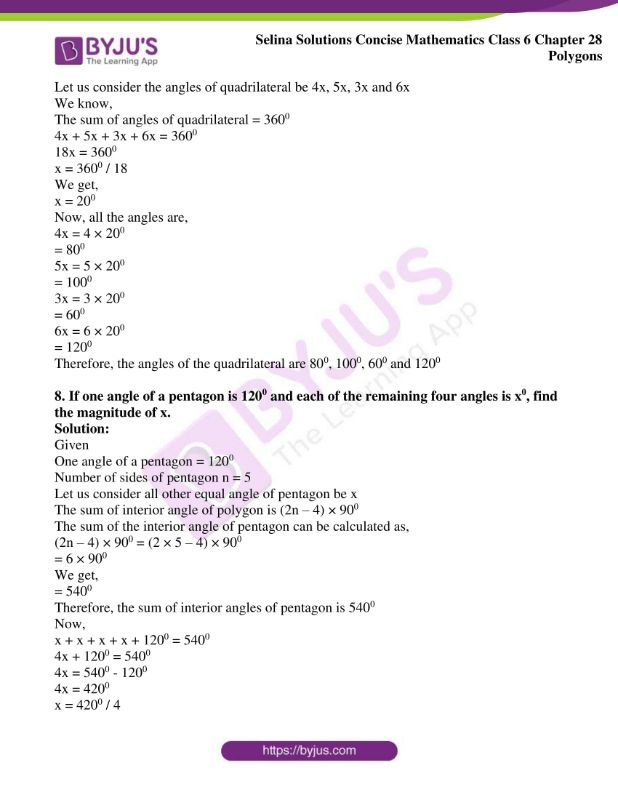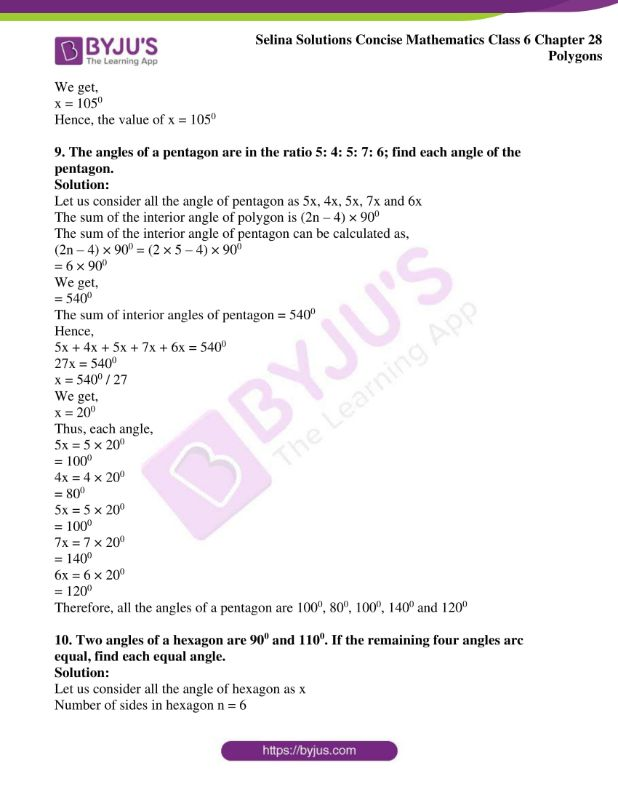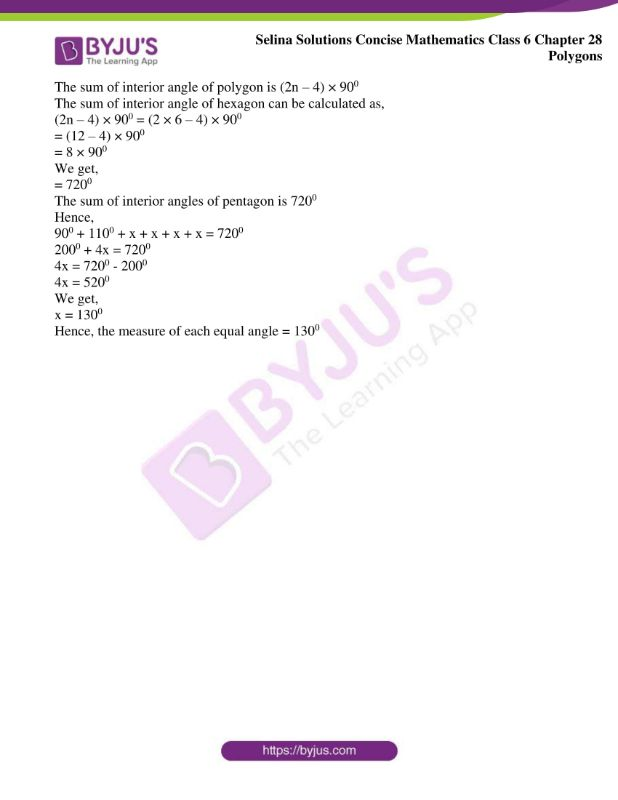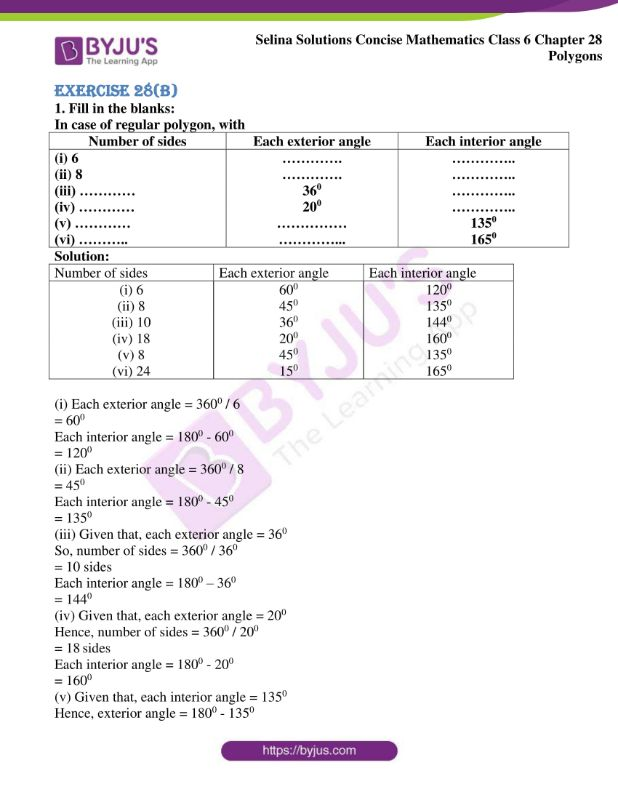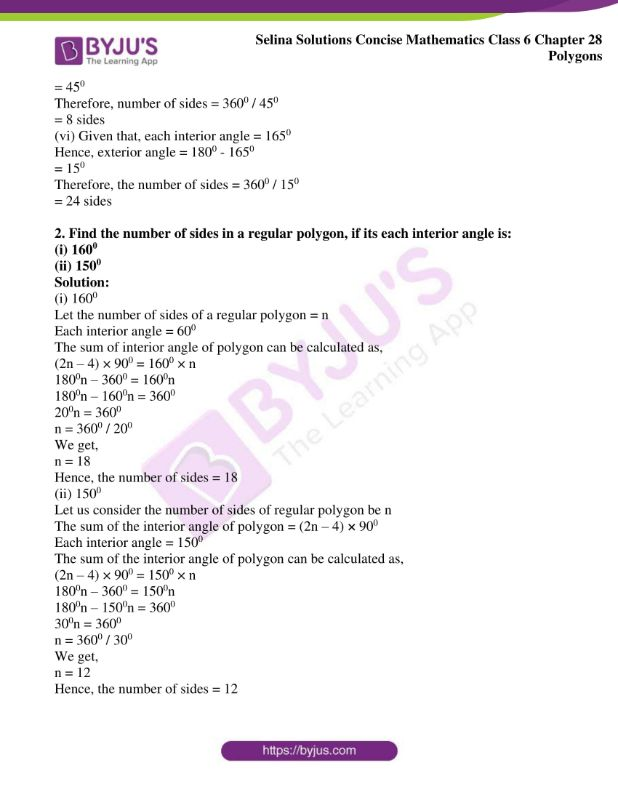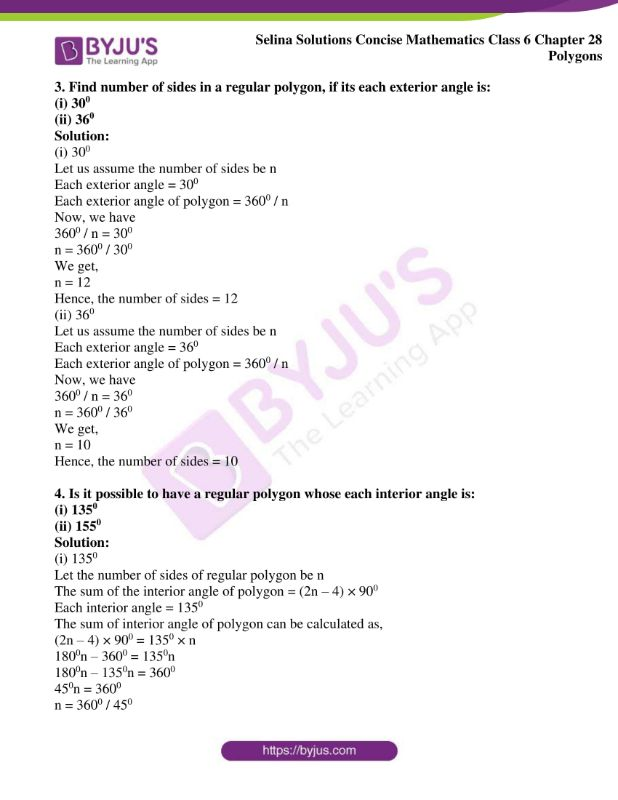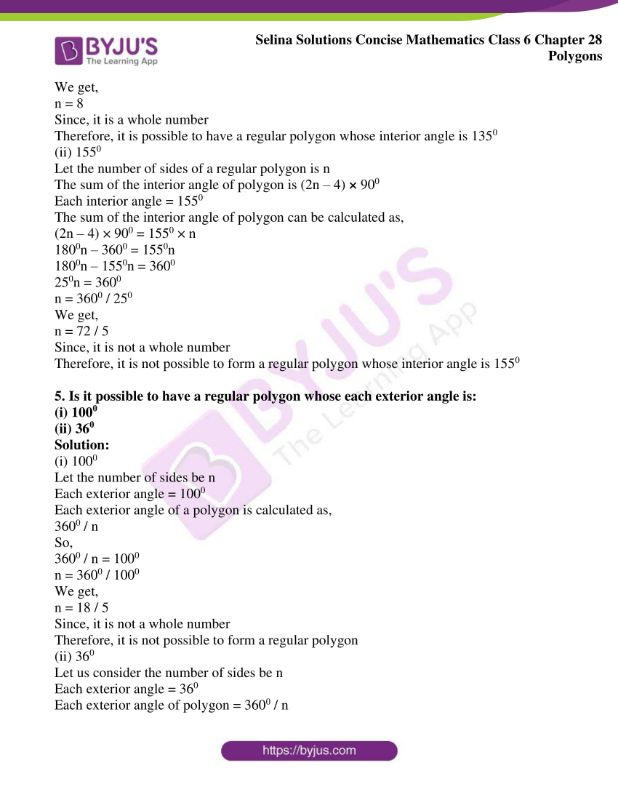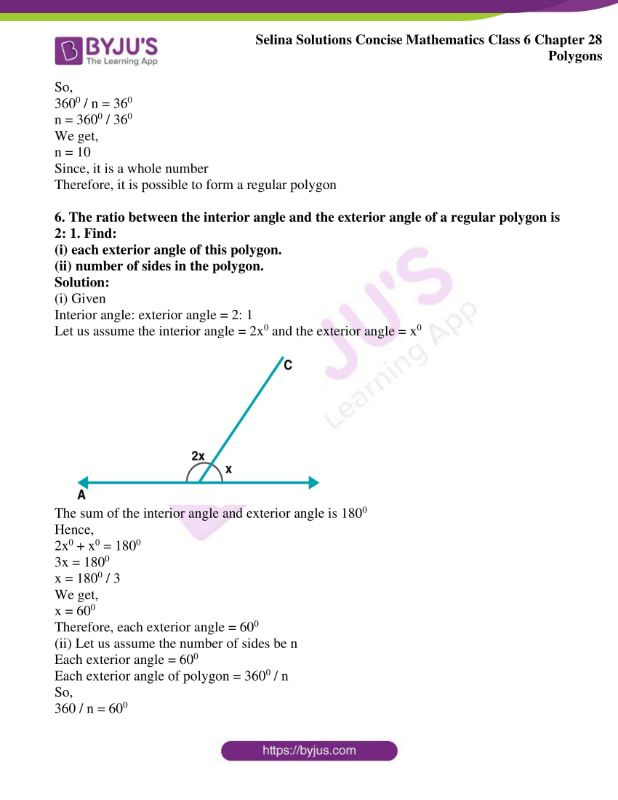### Exercises of Selina Solutions Concise Mathematics Class 6 Chapter 28 Polygons

Exercise 28(A) Solutions

Exercise 28(B) Solutions

## Access Selina Solutions Concise Mathematics Class 6 Chapter 28 Polygons

Exercise 28(A)

1. State, which of the following are polygons: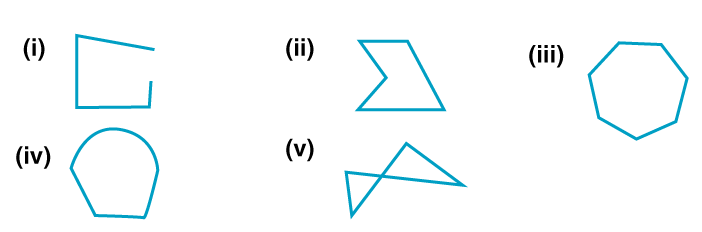Solution:

(i) The given figure is not closed.

Hence, the figure is not a polygon.

(ii) The given figure is closed.

Hence, the figure is a polygon.

(iii) The given figure is closed.

Hence, the figure is a polygon.

(iv) In the given figure, one of the sides is an arc.

Hence, the figure is not polygon.

(v) The side intersects each other in the given figure.

Hence, the figure is not polygon.

2. Find the sum of interior angles of a polygon with:

(i) 9 sides

(ii) 13 sides

(iii) 16 sides

Solution:

(i) 9 sides

Number of sides n = 9

The Sum of interior angles of polygon = (2n – 4) × 900

= (2 × 9 – 4) × 900

= (18 – 4) × 900

= 14 × 900

We get,

= 12600

(ii) 13 sides

Number of sides n = 13

The sum of interior angles of polygon = (2n -4) × 900

= (2 × 13 – 4) × 900

= (26 – 4) × 900

= 22 × 900

We get,

= 19800

(iii) 16 sides

Number of sides n = 16

The sum of interior angles of polygon = (2n – 4) × 900

= (2 × 16 – 4) × 900

= (32 – 4) × 900

= 28 × 900

We get,

= 25200

3. Find the number of sides of a polygon, if the sum of its interior angles is:

(i) 14400

(ii) 16200

Solution:

(i) 14400

The sum of interior angles of polygon = 14400

Let the number of sides = n

The sum of interior angle of polygon is (2n – 4) × 900

The side of polygon can be calculated as,

(2n – 4) × 900 = 14400

2n – 4 = 14400 / 900

2(n – 2) = 14400 / 900

n – 2 = 14400 / (2 × 900)

On further calculation, we get

n – 2 = 160 / 2

We get,

n – 2 = 8

n = 8 + 2

n = 10

Hence, the side of polygon = 10

(ii) 16200

Given

The sum of interior angles of polygon = 16200

Let number of sides = n

The sum of interior angles of polygon = (2n – 4) × 900

The side of polygon can be calculated as,

(2n – 4) × 900 = 16200

2(n – 2) × 900 = 16200

n – 2 = 16200 / (2 × 900)

n – 2 = 8100 / 900

We get,

n – 2 = 9

n = 9 + 2

n = 11

Hence, the side of polygon = 11

4. Is it possible to have a polygon, whose sum of interior angles is 10300.

Solution:

Given

The sum of interior angles of polygon = 10300

Let us consider the number of sides = n

The sum of interior angle of polygon = (2n – 4) × 900

The side of polygon is calculated as,

(2n – 4) × 900 = 10300

2(n – 2)= 10300 / 900

On further calculation, we get

(n – 2) = 10300 / (2 × 900)

(n – 2) = 1030 / 180

n = 5.72 + 2

n = 7.72

which is not a whole number.

Therefore, it is not a polygon, whose sum of interior angles is 10300

5. (i) If all the angles of a hexagon arc equal, find the measure of each angle.

(ii) If all the angles of an octagon are equal, find the measure of each angle.

Solution:

(i) Number of sides of polygon n = 6

Let us consider each angle be = x0

We know,

The sum of interior angles of hexagon = 6x0

The sum of interior angle of polygon = (2n – 4) × 900

The sum of the interior angles of polygon can be calculated as,

(2n – 4) × 900 = Sum of angles

(2 × 6 – 4) × 900 = 6x0

(12 – 4) × 900 = 6x0

6x0 = 8 × 900

x0 = (8 × 900) / 6

We get,

x = 1200

Therefore, each angle of hexagon = 1200

(ii) Number of sides of octagon n = 8

Let us consider each angle be = x0

We know that,

The sum of interior angles of octagon = 8x0

The sum of interior angles of polygon = (2n – 4) × 900

The sum of interior angles of polygon can be calculated as,

(2n – 4) × 900 = Sum of angles

(2n – 4) × 900 = 8x0

(2 × 8 – 4) × 900 = 8x0

12 × 900 = 8x0

8x0 = 12 × 900

x0 = (12 × 900) / 8

We get,

x0 = 1350

Therefore, each angle of octagon = 1350

6. One angle of a quadrilateral is 900 and all other angles are equal; find each equal angle

Solution:

Let us consider all the three equal angle of a quadrilateral be x0

The sum of angles of a quadrilateral = 3600

x + x + x + 900 = 3600

3x + 900 = 3600

3x = 3600 – 900

3x = 2700

x = 2700 / 3

We get,

x = 900

The measure of each equal angle = 900

7. If angles of quadrilateral are in the ratio 4: 5: 3: 6; find each angle of the quadrilateral.

Solution:

Let us consider the angles of quadrilateral be 4x, 5x, 3x and 6x

We know,

The sum of angles of quadrilateral = 3600

4x + 5x + 3x + 6x = 3600

18x = 3600

x = 3600 / 18

We get,

x = 200

Now, all the angles are,

4x = 4 × 200

= 800

5x = 5 × 200

= 1000

3x = 3 × 200

= 600

6x = 6 × 200

= 1200

Therefore, the angles of the quadrilateral are 800, 1000, 600 and 1200

8. If one angle of a pentagon is 1200 and each of the remaining four angles is x0, find the magnitude of x.

Solution:

Given

One angle of a pentagon = 1200

Number of sides of pentagon n = 5

Let us consider all other equal angle of pentagon be x

The sum of interior angle of polygon is (2n – 4) × 900

The sum of the interior angle of pentagon can be calculated as,

(2n – 4) × 900 = (2 × 5 – 4) × 900

= 6 × 900

We get,

= 5400

Therefore, the sum of interior angles of pentagon is 5400

Now,

x + x + x + x + 1200 = 5400

4x + 1200 = 5400

4x = 5400 – 1200

4x = 4200

x = 4200 / 4

We get,

x = 1050

Hence, the value of x = 1050

9. The angles of a pentagon are in the ratio 5: 4: 5: 7: 6; find each angle of the pentagon.

Solution:

Let us consider all the angle of pentagon as 5x, 4x, 5x, 7x and 6x

The sum of the interior angle of polygon is (2n – 4) × 900

The sum of the interior angle of pentagon can be calculated as,

(2n – 4) × 900 = (2 × 5 – 4) × 900

= 6 × 900

We get,

= 5400

The sum of interior angles of pentagon = 5400

Hence,

5x + 4x + 5x + 7x + 6x = 5400

27x = 5400

x = 5400 / 27

We get,

x = 200

Thus, each angle,

5x = 5 × 200

= 1000

4x = 4 × 200

= 800

5x = 5 × 200

= 1000

7x = 7 × 200

= 1400

6x = 6 × 200

= 1200

Therefore, all the angles of a pentagon are 1000, 800, 1000, 1400 and 1200

10. Two angles of a hexagon are 900 and 1100. If the remaining four angles arc equal, find each equal angle.

Solution:

Let us consider all the angle of hexagon as x

Number of sides in hexagon n = 6

The sum of interior angle of polygon is (2n – 4) × 900

The sum of interior angle of hexagon can be calculated as,

(2n – 4) × 900 = (2 × 6 – 4) × 900

= (12 – 4) × 900

= 8 × 900

We get,

= 7200

The sum of interior angles of pentagon is 7200

Hence,

900 + 1100 + x + x + x + x = 7200

2000 + 4x = 7200

4x = 7200 – 2000

4x = 5200

We get,

x = 1300

Hence, the measure of each equal angle = 1300

Exercise 28(B)

1. Fill in the blanks:

In case of regular polygon, with

 Number of sides Each exterior angle Each interior angle (i) 6 …………. ………….. (ii) 8 …………. ………….. (iii) ………… 360 ………….. (iv) ………… 200 ………….. (v) ………… …………… 1350 (vi) ……….. …………… 1650

Solution:

 Number of sides Each exterior angle Each interior angle (i) 6 600 1200 (ii) 8 450 1350 (iii) 10 360 1440 (iv) 18 200 1600 (v) 8 450 1350 (vi) 24 150 1650

(i) Each exterior angle = 3600 / 6

= 600

Each interior angle = 1800 – 600

= 1200

(ii) Each exterior angle = 3600 / 8

= 450

Each interior angle = 1800 – 450

= 1350

(iii) Given that, each exterior angle = 360

So, number of sides = 3600 / 360

= 10 sides

Each interior angle = 1800 – 360

= 1440

(iv) Given that, each exterior angle = 200

Hence, number of sides = 3600 / 200

= 18 sides

Each interior angle = 1800 – 200

= 1600

(v) Given that, each interior angle = 1350

Hence, exterior angle = 1800 – 1350

= 450

Therefore, number of sides = 3600 / 450

= 8 sides

(vi) Given that, each interior angle = 1650

Hence, exterior angle = 1800 – 1650

= 150

Therefore, the number of sides = 3600 / 150

= 24 sides

2. Find the number of sides in a regular polygon, if its each interior angle is:

(i) 1600

(ii) 1500

Solution:

(i) 1600

Let the number of sides of a regular polygon = n

Each interior angle = 600

The sum of interior angle of polygon can be calculated as,

(2n – 4) × 900 = 1600 × n

1800n – 3600 = 1600n

1800n – 1600n = 3600

200n = 3600

n = 3600 / 200

We get,

n = 18

Hence, the number of sides = 18

(ii) 1500

Let us consider the number of sides of regular polygon be n

The sum of the interior angle of polygon = (2n – 4) × 900

Each interior angle = 1500

The sum of the interior angle of polygon can be calculated as,

(2n – 4) × 900 = 1500 × n

1800n – 3600 = 1500n

1800n – 1500n = 3600

300n = 3600

n = 3600 / 300

We get,

n = 12

Hence, the number of sides = 12

3. Find number of sides in a regular polygon, if its each exterior angle is:

(i) 300

(ii) 360

Solution:

(i) 300

Let us assume the number of sides be n

Each exterior angle = 300

Each exterior angle of polygon = 3600 / n

Now, we have

3600 / n = 300

n = 3600 / 300

We get,

n = 12

Hence, the number of sides = 12

(ii) 360

Let us assume the number of sides be n

Each exterior angle = 360

Each exterior angle of polygon = 3600 / n

Now, we have

3600 / n = 360

n = 3600 / 360

We get,

n = 10

Hence, the number of sides = 10

4. Is it possible to have a regular polygon whose each interior angle is:

(i) 1350

(ii) 1550

Solution:

(i) 1350

Let the number of sides of regular polygon be n

The sum of the interior angle of polygon = (2n – 4) × 900

Each interior angle = 1350

The sum of interior angle of polygon can be calculated as,

(2n – 4) × 900 = 1350 × n

1800n – 3600 = 1350n

1800n – 1350n = 3600

450n = 3600

n = 3600 / 450

We get,

n = 8

Since, it is a whole number

Therefore, it is possible to have a regular polygon whose interior angle is 1350

(ii) 1550

Let the number of sides of a regular polygon is n

The sum of the interior angle of polygon is (2n – 4) × 900

Each interior angle = 1550

The sum of the interior angle of polygon can be calculated as,

(2n – 4) × 900 = 1550 × n

1800n – 3600 = 1550n

1800n – 1550n = 3600

250n = 3600

n = 3600 / 250

We get,

n = 72 / 5

Since, it is not a whole number

Therefore, it is not possible to form a regular polygon whose interior angle is 1550

5. Is it possible to have a regular polygon whose each exterior angle is:

(i) 1000

(ii) 360

Solution:

(i) 1000

Let the number of sides be n

Each exterior angle = 1000

Each exterior angle of a polygon is calculated as,

3600 / n

So,

3600 / n = 1000

n = 3600 / 1000

We get,

n = 18 / 5

Since, it is not a whole number

Therefore, it is not possible to form a regular polygon

(ii) 360

Let us consider the number of sides be n

Each exterior angle = 360

Each exterior angle of polygon = 3600 / n

So,

3600 / n = 360

n = 3600 / 360

We get,

n = 10

Since, it is a whole number

Therefore, it is possible to form a regular polygon

6. The ratio between the interior angle and the exterior angle of a regular polygon is 2: 1. Find:

(i) each exterior angle of this polygon.

(ii) number of sides in the polygon.

Solution:

(i) Given

Interior angle: exterior angle = 2: 1

Let us assume the interior angle = 2x0 and the exterior angle = x0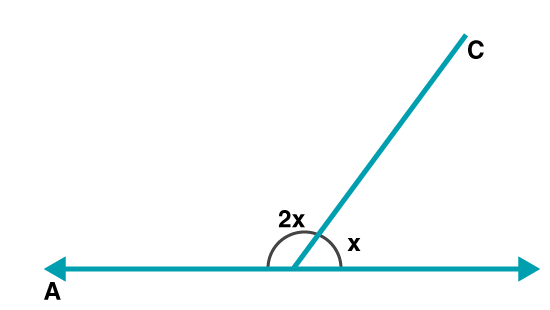The sum of the interior angle and exterior angle is 1800

Hence,

2x0 + x0 = 1800

3x = 1800

x = 1800 / 3

We get,

x = 600

Therefore, each exterior angle = 600

(ii) Let us assume the number of sides be n

Each exterior angle = 600

Each exterior angle of polygon = 3600 / n

So,

360 / n = 600

n = 3600 / 600

We get,

n = 6

Hence, the number of sides = 6